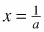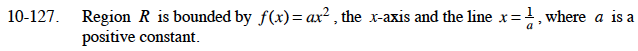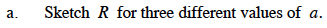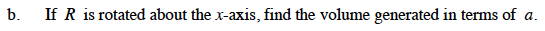### Home > CALC > Chapter 10 > Lesson 10.3.2 > Problem10-127

10-127.
1. Region R is bounded by f(x) = ax2 , the x-axis and the line, where a is a positive constant. Homework Help ✎

1. Sketch R for three different values of a.

2. If R is rotated about the x-axis, find the volume generated in terms of a.$\int_0^{1/a}\pi(ax^2)^2dx$